# sbiosobol

Perform global sensitivity analysis by computing first- and total-order Sobol indices (requires Statistics and Machine Learning Toolbox)

## Syntax

``sobolResults = sbiosobol(modelObj,params,observables)``
``sobolResults = sbiosobol(modelObj,scenarios,observables)``
``sobolResults = sbiosobol(modelObj,params,observables,Name,Value)``

## Description

example

````sobolResults = sbiosobol(modelObj,params,observables)` performs global sensitivity analysis  on a SimBiology model `modelObj` by decomposing the variances of `observables` with respect to the sensitivity inputs `params`.```
````sobolResults = sbiosobol(modelObj,scenarios,observables)` uses samples from `scenarios`, a `SimBiology.Scenarios` object, to perform the analysis.```

example

````sobolResults = sbiosobol(modelObj,params,observables,Name,Value)` uses additional options specified by one or more name-value pair arguments.```

## Examples

collapse all

`sbioloadproject tumor_growth_vpop_sa.sbproj`

Get a variant with the estimated parameters and the dose to apply to the model.

```v = getvariant(m1); d = getdose(m1,'interval_dose');```

Get the active configset and set the tumor weight as the response.

```cs = getconfigset(m1); cs.RuntimeOptions.StatesToLog = 'tumor_weight';```

Simulate the model and plot the tumor growth profile.

`sbioplot(sbiosimulate(m1,cs,v,d));`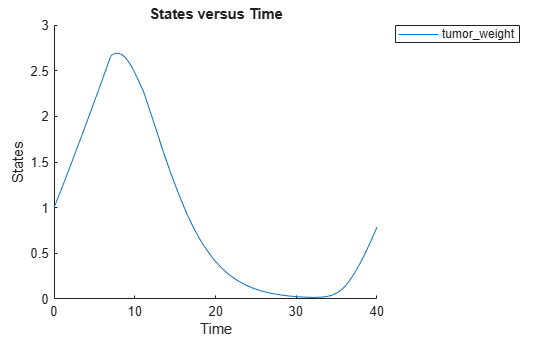Perform global sensitivity analysis (GSA) on the model to find the model parameters that the tumor growth is sensitive to.

First, retrieve model parameters of interest that are involved in the pharmacodynamics of the tumor growth. Define the model response as the tumor weight.

```modelParamNames = {'L0','L1','w0','k1','k2'}; outputName = 'tumor_weight';```

Then perform GSA by computing the first- and total-order Sobol indices using `sbiosobol`. Set `'ShowWaitBar'` to `true` to show the simulation progress. By default, the function uses 1000 parameter samples to compute the Sobol indices .

```rng('default'); sobolResults = sbiosobol(m1,modelParamNames,outputName,'Variants',v,'Doses',d,'ShowWaitBar',true)```
```sobolResults = Sobol with properties: Time: [444x1 double] SobolIndices: [5x1 struct] Variance: [444x1 table] ParameterSamples: [1000x5 table] Observables: {'[Tumor Growth Model].tumor_weight'} SimulationInfo: [1x1 struct] ```

You can change the number of samples by specifying the `'NumberSamples'` name-value pair argument. The function requires a total of `(number of input parameters + 2) * NumberSamples` model simulations.

Show the mean model response, the simulation results, and a shaded region covering 90% of the simulation results.

`plotData(sobolResults);`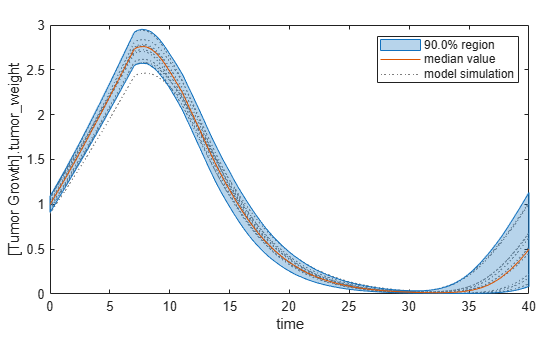You can adjust the quantile region to a different percentage by specifying `'Alphas' `for the lower and upper quantiles of all model responses. For instance, an alpha value of 0.1 plots a shaded region between the `100 * alpha` and `100 * (1 - alpha)` quantiles of all simulated model responses.

`plotData(sobolResults,'Alphas',0.1);`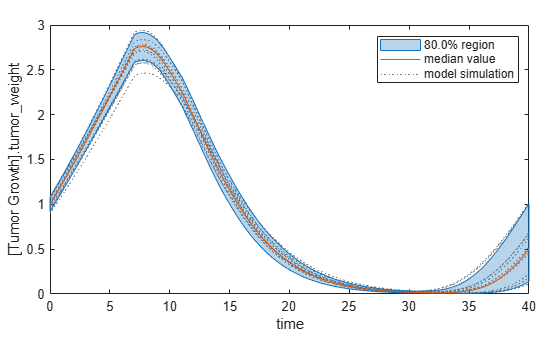Plot the time course of the first- and total-order Sobol indices.

```h = plot(sobolResults); % Resize the figure. h.Position(:) = [100 100 1280 800];```The first-order Sobol index of an input parameter gives the fraction of the overall response variance that can be attributed to variations in the input parameter alone. The total-order index gives the fraction of the overall response variance that can be attributed to any joint parameter variations that include variations of the input parameter.

From the Sobol indices plots, parameters `L1` and `w0` seem to be the most sensitive parameters to the tumor weight before the dose was applied at t = 7. But after the dose is applied, `k1` and `k2` become more sensitive parameters and contribute most to the after-dosing stage of the tumor weight. The total variance plot also shows a larger variance for the after-dose stage at t > 35 than for the before-dose stage of the tumor growth, indicating that `k1` and `k2` might be more important parameters to investigate further. The fraction of unexplained variance shows some variance at around t = 33, but the total variance plot shows little variance at t = 33, meaning the unexplained variance could be insignificant. The fraction of unexplained variance is calculated as 1 - (sum of all the first-order Sobol indices), and the total variance is calculated using `var(response)`, where `response` is the model response at every time point.

You can also display the magnitudes of the sensitivities in a bar plot. Darker colors mean that those values occur more often over the whole time course.

`bar(sobolResults);`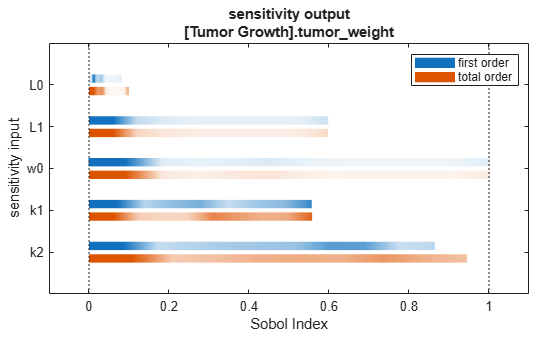You can specify more samples to increase the accuracy of the Sobol indices, but the simulation can take longer to finish. Use `addsamples` to add more samples. For example, if you specify 1500 samples, the function performs `1500 * (2 + number of input parameters)` simulations.

```gsaMoreSamples = addsamples(gsaResults,1500) ```

The SimulationInfo property of the result object contains various information for computing the Sobol indices. For instance, the model simulation data (SimData) for each simulation using a set of parameter samples is stored in the `SimData` field of the property. This field is an array of `SimData` objects.

`sobolResults.SimulationInfo.SimData`
``` SimBiology SimData Array : 1000-by-7 Index: Name: ModelName: DataCount: 1 - Tumor Growth Model 1 2 - Tumor Growth Model 1 3 - Tumor Growth Model 1 ... 7000 - Tumor Growth Model 1 ```

You can find out if any model simulation failed during the computation by checking the `ValidSample` field of `SimulationInfo`. In this example, the field shows no failed simulation runs.

`all(sobolResults.SimulationInfo.ValidSample)`
```ans = 1x7 logical array 1 1 1 1 1 1 1 ```

`SimulationInfo.ValidSample` is a table of logical values. It has the same size as `SimulationInfo.SimData`. If `ValidSample` indicates that any simulations failed, you can get more information about those simulation runs and the samples used for those runs by extracting information from the corresponding column of `SimulationInfo.SimDat`a. Suppose that the fourth column contains one or more failed simulation runs. Get the simulation data and sample values used for that simulation using `getSimulationResults`.

`[samplesUsed,sd,validruns] = getSimulationResults(sobolResults,4);`

You can add custom expressions as observables and compute Sobol indices for the added observables. For example, you can compute the Sobol indices for the maximum tumor weight by defining a custom expression as follows.

```% Suppress an information warning that is issued during simulation. warnSettings = warning('off', 'SimBiology:sbservices:SB_DIMANALYSISNOTDONE_MATLABFCN_UCON'); % Add the observable expression. sobolObs = addobservable(sobolResults,'Maximum tumor_weight','max(tumor_weight)','Units','gram');```

Plot the computed simulation results showing the 90% quantile region.

```h2 = plotData(sobolObs); h2.Position(:) = [100 100 1280 800];```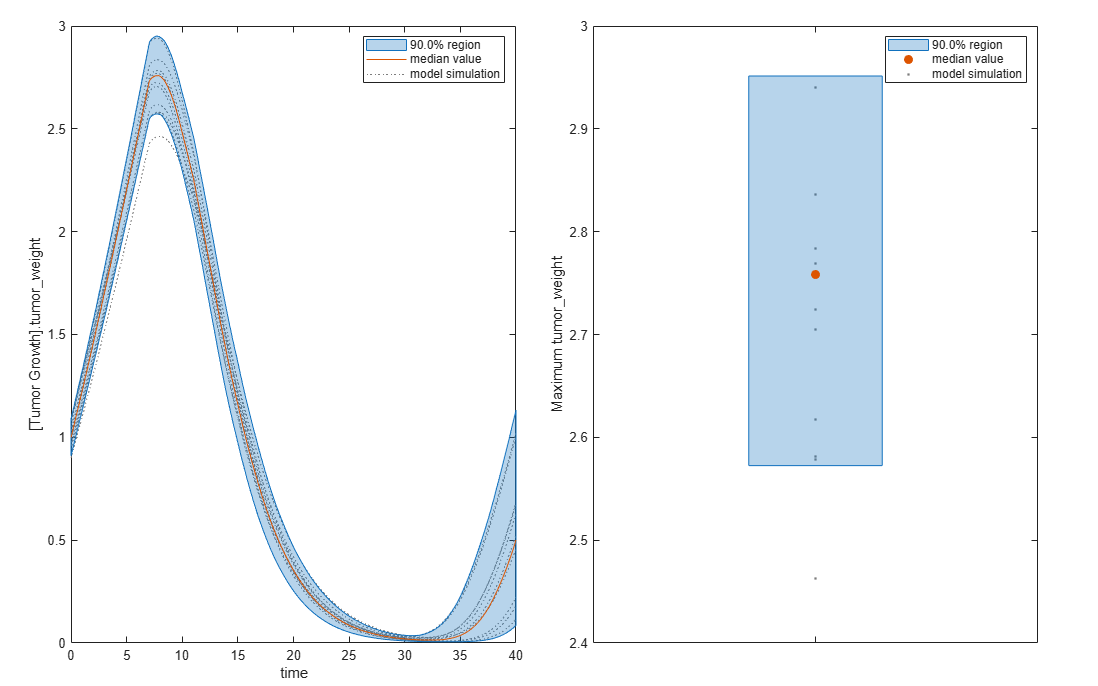You can also remove the observable by specifying its name.

`gsaNoObs = removeobservable(sobolObs,'Maximum tumor_weight');`

Restore the warning settings.

`warning(warnSettings);`

## Input Arguments

collapse all

SimBiology model, specified as a SimBiology `model object`.

Names of model parameters, species, or compartments, specified as a character vector, string, string vector, or cell array of character vectors.

Example: `["k1","k2"]`

Data Types: `char` | `string` | `cell`

Model responses, specified as a character vector, string, string vector, or cell array of character vectors. Specify the names of species, parameters, compartments, or `observables`.

Example: `["tumor_growth"]`

Data Types: `char` | `string` | `cell`

Source for drawing samples, specified as a `SimBiology.Scenarios` object.

• You must combine entries of the object elementwise.

• Entries must be independent random variables. If there are multiple entries, they must be uncorrelated.

• `SamplingMethod` of any entry must not be `copula`.

### Name-Value Arguments

Specify optional comma-separated pairs of `Name,Value` arguments. `Name` is the argument name and `Value` is the corresponding value. `Name` must appear inside quotes. You can specify several name and value pair arguments in any order as `Name1,Value1,...,NameN,ValueN`.

Example: ```sobolResults = sbiosobol(modelObj,params,observables,'ShowWaitbar',true)``` specifies to show a simulation progress bar.

Parameter bounds, specified as a numeric matrix with two columns. The first column contains the lower bounds and the second column contains the upper bounds. The number of rows must be equal to the number of parameters in `params`.

If a parameter has a nonzero value, the default bounds are ±10% of the value. If the parameter value is zero, the default bounds are `[0 1]`.

Example: `[0.5 5]`

Data Types: `double`

Doses to use during model simulations, specified as a `ScheduleDose` or `RepeatDose` object or a vector of dose objects.

Variants to apply before model simulations, specified as a variant object or vector of variant objects.

When you specify multiple variants with duplicate specifications for a property's value, the last occurrence for the property value in the array of variants is used during simulation.

Number of samples to compute Sobol indices, specified as the comma-separated pair consisting of `'NumberSamples'` and a positive integer. The function requires ```(number of input params + 2) * NumberSamples``` model simulations to compute the first- and total-order Sobol indices.

Data Types: `double`

Probability distributions used to draw samples, specified as a `prob.ProbabilityDistribution` object or vector of these objects. Specify a scalar `prob.ProbabilityDistribution` or vector of length N, where N is the number of input parameters. You can create distribution objects to sample from various distributions, such as uniform, normal, or lognormal distributions, using `makedist` (Statistics and Machine Learning Toolbox).

If you specify a scalar `prob.ProbabilityDistribution` object, and there are multiple input parameters, `sbiosobol` uses the same distribution object to draw samples for each parameter.

You cannot specify this argument together with Bounds.

You cannot specify this argument when a `SimBiology.Scenarios` object is an input.

Method to generate parameter samples, specified as a character vector or string. Valid options are:

• `'Sobol'` — Use the low-discrepancy Sobol sequence to generate samples.

• `'Halton'` — Use the low-discrepancy Halton sequence to generate samples.

• `'lhs'` — Use the low-discrepancy Latin hypercube samples.

• `'random'` — Use uniformly distributed random samples.

Data Types: `char` | `string`

Options for the sampling method, specified as a scalar struct. The options differ depending on the sampling method: `sobol`, `halton`, or `lhs`.

For `sobol` and `halton`, specify each field name and value of the structure according to each name-value argument of the `sobolset` (Statistics and Machine Learning Toolbox) or `haltonset` (Statistics and Machine Learning Toolbox) function. SimBiology uses the default value of `1` for the `Skip` argument for both methods. For all other name-value arguments, the software uses the same default values of `sobolset` or `haltonset`. For instance, set up a structure for the `Leap` and `Skip` options with nondefault values as follows.

```s1.Leap = 50; s1.Skip = 0;```

For `lhs`, there are three samplers that support different sampling options.

• If you specify a covariance matrix, SimBiology uses `lhsnorm` (Statistics and Machine Learning Toolbox) for sampling. `SamplingOptions` argument is not allowed.

• Otherwise, use the field name `UseLhsdesign` to select a sampler.

• If the value is `true`, SimBiology uses `lhsdesign` (Statistics and Machine Learning Toolbox). You can use the name-value arguments of `lhsdesign` to specify the field names and values.

• If the value is `false` (default), SimBiology uses a nonconfigurable Latin hypercube sampler that is different from `lhsdesign`. This sampler does not require Statistics and Machine Learning Toolbox™. `SamplingOptions` cannot contain any other options, except `UseLhsdesign`.

For instance, set up a structure to use `lhsdesign` with the `Criterion` and `Iterations` options.

```s2.UseLhsdesign = true; s2.Criterion = "correlation"; s2.Iterations = 10;```

You cannot specify this argument when a `SimBiology.Scenarios` object is an input.

Simulation stop time, specified as a nonnegative scalar. If you specify neither `StopTime` nor `OutputTimes`, the function uses the stop time from the active configuration set of the model. You cannot specify both `StopTime` and `OutputTimes`.

Data Types: `double`

Simulation output times, specified as the comma-separated pair consisting of `'OutputTimes'` and a numeric vector. The function computes the Sobol indices at these output time points. You cannot specify both `StopTime` and `OutputTimes`. By default, the function uses the output times of the first model simulation.

Example: `[0 1 2 3.5 4 5 5.5]`

Data Types: `double`

Flag to run model simulations in parallel, specified as `true` or `false`. When the value is `true` and Parallel Computing Toolbox™ is available, the function runs simulations in parallel.

Data Types: `logical`

Flag to turn on model acceleration, specified as `true` or `false`.

Data Types: `logical`

Method for interpolation of model responses to a common set of output times, specified as a character vector or string. The valid options follow.

• `"interp1q"` — Use the `interp1q` function.

• Use the `interp1` function by specifying one of the following methods:

• `"nearest"`

• `"linear"`

• `"spline"`

• `"pchip"`

• `"v5cubic"`

• `"zoh"` — Specify zero-order hold.

Data Types: `char` | `string`

Flag to show the progress of model simulations by displaying a progress bar, specified as the comma-separated pair consisting of `'ShowWaitbar'` and `true` or `false`. By default, no wait bar is displayed.

Data Types: `logical`

## Output Arguments

collapse all

Results containing the first- and total-order Sobol indices, returned as a `SimBiology.gsa.Sobol` object. The object also contains the parameter sample values and model simulation data used to compute the Sobol indices.

The results object can contain a significant amount of simulation data (SimData). The size of the object exceeds ```(1 + number of observables) * number of output time points * (2 + number of parameters) * number of samples * 8``` bytes. For example, if you have one observable, 500 output time points, 8 parameters, and 100,000 samples, the object size is `(1 + 1) * 500 * (2 + 8) * 100000 * 8` bytes = 8 GB. If you need to save such large objects, use this syntax:

`save(fileName,variableName,'-v7.3');`
For details, see MAT-file version.

collapse all

### Saltelli Method to Compute Sobol Indices

`sbiosobol` implements the Saltelli method  to compute Sobol indices.

Consider a SimBiology model response Y expressed as a mathematical model $Y=f\left({X}_{1},{X}_{2},{X}_{3},...,{X}_{k}\right)$, where Xi is a model parameter and `i = 1,…,k`.

The first-order Sobol index (Si) gives the fraction of the overall response variance `V(Y)` that can be attributed to variations in Xi alone. Si is defined as follows.

`${S}_{i}=\frac{{V}_{{X}_{i}}\left({E}_{{X}_{\sim i}}\left(Y|{X}_{i}\right)\right)}{V\left(Y\right)}$`

The total-order Sobol index (STi) gives the fraction of the overall response variance `V(Y)` that can be attributed to any joint parameter variations that include variations of Xi. STi is defined as follows.

`${S}_{Ti}=1-\frac{{V}_{{X}_{\sim i}}\left({E}_{{X}_{i}}\left(Y|{X}_{\sim i}\right)\right)}{V\left(Y\right)}=\frac{{E}_{{X}_{\sim i}}\left({V}_{{X}_{i}}\left(Y|{X}_{\sim i}\right)\right)}{V\left(Y\right)}$`

To compute individual values for Y corresponding to samples of parameters X1, X1, …, Xk, consider two independent sampling matrices A and B.

`$A=\left(\begin{array}{cccc}{X}_{11}& {X}_{12}& ...& {X}_{1k}\\ {X}_{21}& {X}_{22}& ...& {X}_{2k}\\ ...& ...& ...& ...\\ {X}_{n1}& {X}_{n2}& ...& {X}_{nk}\end{array}\right)$`

`$B=\left(\begin{array}{cccc}{X}_{11}^{\text{'}}& {X}_{12}^{\text{'}}& ...& {X}_{1k}^{\text{'}}\\ {X}_{21}^{\text{'}}& {X}_{22}^{\text{'}}& ...& {X}_{2k}^{\text{'}}\\ ...& ...& ...& ...\\ {X}_{n1}^{\text{'}}& {X}_{n2}^{\text{'}}& ...& {X}_{nk}^{\text{'}}\end{array}\right)$`

n is the sample size. Each row of the matrices A and B corresponds to one parameter sample set, which is a single realization of model parameter values.

Estimates for Si and STi are obtained from model simulation results using sample values from the matrices A, B, and ${A}_{B}^{i}$, which is a matrix where all columns are from A except the ith column, which is from B for ```i = 1, 2, …, params```.

`${A}_{B}^{i}=\left(\begin{array}{cccccc}{X}_{11}& {X}_{12}& ...& {X}_{1i}^{\text{'}}& ...& {X}_{1k}\\ {X}_{21}& {X}_{22}& ...& {X}_{2i}^{\text{'}}& ...& {X}_{2k}\\ ...& ...& ...& ...& ...& ...\\ {X}_{n1}& {X}_{n2}& ...& {X}_{ni}^{\text{'}}& ...& {X}_{nk}\end{array}\right)$`

The formulas to approximate the first- and total-order Sobol indices are as follows.

`${\stackrel{^}{S}}_{i}=\frac{\frac{1}{n}\sum _{j=1}^{n}f{\left(B\right)}_{j}\left(f{\left({A}_{B}^{i}\right)}_{j}-f{\left(A\right)}_{j}\right)}{V\left(Y\right)}$`

`${\stackrel{^}{S}}_{Ti}=\frac{\frac{1}{2n}\sum _{j=1}^{n}{\left(f{\left(A\right)}_{j}-f{\left({A}_{B}^{i}\right)}_{j}\right)}^{2}}{V\left(Y\right)}$`

`f(A)`, `f(B)`, and $f{\left({A}_{B}^{i}\right)}_{j}$ are the model simulation results using the parameter sample values from matrices A, B, and ${A}_{B}^{i}$.

The matrix A corresponds to the `ParameterSamples` property of the Sobol results object (`resultsObj.ParameterSamples`). The matrix B corresponds to the `SupportSamples` property (`resultsObj.SimulationInfo.SupportSamples`).

The ${A}_{B}^{i}$ matrices are stored in the `SimData` structure of the `SimulationInfo` property (`resultsObj.SimulationInfo.SimData`). The size of `SimulationInfo.SimData` is ```NumberSamples-by-params + 2```, where NumberSamples is the number of samples and param is the number of input parameters. The number of columns is `2 + params` because the first column of `SimulationInfo.SimData` contains the model simulation results using the sample matrix A. The second column contains simulation results using `SupportSamples`, which is another sample matrix B. The rest of the columns contain simulation results using ${A}_{B}^{1}$, ${A}_{B}^{2}$, …, ${A}_{B}^{i}$, …, ${A}_{B}^{params}$. See `getSimulationResults` to retrieve the model simulation results and samples for a specified ith index (${A}_{B}^{i}$) from the `SimulationInfo.SimData` array.

 Saltelli, Andrea, Paola Annoni, Ivano Azzini, Francesca Campolongo, Marco Ratto, and Stefano Tarantola. “Variance Based Sensitivity Analysis of Model Output. Design and Estimator for the Total Sensitivity Index.” Computer Physics Communications 181, no. 2 (February 2010): 259–70. https://doi.org/10.1016/j.cpc.2009.09.018.

## SupportGet trial now